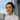# Shopify Liquid divided_by giving zero

Setting up Liquid for server-side rendering for an app and for some reason, a simple arithmetic operation is incorrectly yielding the value zero? I recently met with the same problem with Shopify Liquid divided_by giving zero. The solution is trivial, but the problem could take you (like it did for me) about 20 minutes to debug. The problem? Rounding.

Here is an example of a page that is supposed to display a discount percentage value, which is calculated using Liquid:

{% if sale_price != "" %} {% assign discount = price | minus:sale_price %} {{discount | divided_by:price | times:100 | round}} percent {% else %} 0 {% endif %}

So the logic goes, if sale_price has a value, then compute discount in percentage and show it. Otherwise, show the value 0. Simple, right?

Yes. But when I tried rendering this page with discount=21 and price=53, the computed value that was shown on the page turned out to be “0 percent” instead of “39 percent” as it should have been!

Why?

To debug, I tried rendering only parts of the Liquid markup:

{{discount | divided_by:price | times:100 | round}} percent

{{discount | divided_by:price }} percent

{{discount | divided_by:53 }} percent

{{21 | divided_by:price }} percent

{{discount}} percent

{{price}} percent

From trying these combinations, and after trying to type-coerce the values to numbers by doing `{% discount = discount | plus:1 %}` without a new outcome, I figured that it might be something to do with rounding

I calculated 21/53 on my laptop and it yielded the value 0.39. Zero point three nine… zero… hmm. Could the Liquid markup be rounding values down before the next operator is read and executed? I tested my hypothesis by doing `times` before `divided_by`:

{{discount | divided_by:price | times:100 | round}} percent

{{discount | times:100 | divided_by:price | round}} percent

The bottom line of markup works! Stepwise, the value is computed as such:

21 —> 21*100 = 2100 —> 2100/53 = 39.6 —> 39.6 round = 39 —> 39 percent

Whereas when `divided_by` was put ahead of `times`, it went like this:

21 —> 21/53 = 0.39 ==> auto round to 0 —> 0*100 = 0 —> 0 round = 0 —> 0 percent

So there you go. Apparently Shopify Liquid will round numerical values down before the next operator gets to work on the value, or at least something is happening to that effect. Why? I have no idea. Just another quirk of a programming language to know.

### What is Shopify Liquid?

Liquid is an open-source template language originally developed by Shopify for their internal use. I have several issues with the choices they made with naming operators, but generally speaking, it gets the job done.If something made you think, I would love to know.
email me  |  give coffee